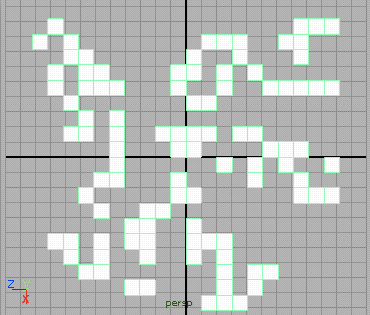Cellular Automata PolyPlanes

Introduction

The tutorial, "Algorithmic Form: Basic CA Python Classes", presented two python classes that implement the logic that underpins the classes developed in this tutorial. The two classes presented in listing 1, CellularPlanes.py, enable a cellular automata to be modelled in Maya as a grid of instanced shapes - figure 1.Figure 1 - 20 by 20 cells [click to play]

The MayaCell Class

This is a subclass of AbstractAutomata.Cell. It has four class variables that are shared by all instances of the class. For efficiency, each instance of MayaCell uses Maya's instance() proc to create a "copy" of single "master" object ie.

self.tformNode = mc.instance(MayaCell.shape)

By default, the master shape is a Maya polyPlane and the attribute that responds to the state of a MayaCell is the visibility of the shape. MayaCell overrides the __init__ method of its super class in order to create a Maya instance and to set its pivot point.

def __init__(self, chance):
# Call the super class init method
CA.Cell.__init__(self, chance)
self.tformNode = mc.instance(MayaCell.shape)
mc.xform(self.tformNode, piv = MayaCell.pivot)

Setting the pivot is of no importance to a polyPlane but it is important when other Maya shapes are used. For example, before an instance of the MayaAutomata class is created the class variables of MayaCell can be assigned new values ie.

mc.delete(MayaCell.shape)
MayaCell.shape = mc.polyCube(w = 0.8, h = 0.8, d = 0.8)
MayaCell.attrName = ".scaleY"
MayaCell.attrIncr = 1
MayaCell.pivot = (0, -0.4, 0)

MayaCell has been written so that a variety of graphics can be produced easily. For example, figure 2 shows the effect of using a polyCubes whose scaleY attribute is "driven" by the number of times a cell was "alive" during 10 generations of the automata.Figure 2

The setState() method enables a variety of potentially interesting visual effects to be easily achieved (figures 3 & 4). If the reader wishes the Maya shape that represents a cell to have more than one attribute effected by a cell's state they should create their own subclass of AbstractAutomata.Cell.

The MayaAutomata Class

The class ensures that instances of MayaCell, rather than the default Cell class are used for the automata ie.

# This overrides the base class so that an instance
# of our custom class can be used by the automata. It
# is called by the Automata.__init__() method.
def getACell(self, chanceOfLife):
cell = MayaCell(chanceOfLife)
MayaAutomata.listOftNodes.append(cell.tformNode)
return cell

The class is responsible for laying out the Maya shapes that represent the cells of the automata. Although the initGraphics() method lays out the shapes in a grid there is no reason why they cannot be positioned in more interesting configurations. For example, figure 5 used this slightly modified version of the method.

def initGraphics(self):
count = 0

angle = 360.0/self.rows
print "\n"
print angle

for i in range(self.cols):
x = startAt
#y = 0
z = startAt + i
for j in range(self.rows):
cell = self.cells[i][j]
mc.xform(cell.tformNode, piv = (0, -radius, 0),
ro = (0, 0, j * angle),
mc.setAttr((MayaCell.shape + ".visibility"), 0)
MayaAutomata.listOftNodes.append(MayaCell.shape)
mc.group(MayaAutomata.listOftNodes)Figure 5 - 20 by 20 cells [click to play]

The classes have been implemented on the assumption the reader wishes to animate the cells over an arbitary number of generations ie. frames of animation.

Running the Automata

Execute code shown below in Maya's script window - ensure the Python tab is active!
Welcome to cellular automata.

import maya.cmds as mc
import random
import CellularPlanes
# Use reload if CellularPlanes.py is modified

# To replace the default shape with one of your choosing
# the next 5 lines of code must be un-commented
#mc.delete(MayaCell.shape)
#CellularPlanes.MayaCell.shape = mc.polyCube(w = 0.8, h = 0.8, d = 0.8)
#CellularPlanes.MayaCell.attrName = ".scaleY"
#CellularPlanes.MayaCell.attrIncr = 1
#CellularPlanes.MayaCell.pivot = (0, -0.4, 0)

# Lock the random number generator
random.seed(1)

# Create the automata with 16 cells along
# the X axis and 10 cells along the Z axes
automata = CellularPlanes.MayaAutomata(16, 10, 0.5)

generations = 10
for n in range(generations):
mc.currentTime(n)
automata.nextGeneration()

Listing 1 (CellularPlanes.py)

 import AbstractAutomata as CA import maya.cmds as mc class MayaCell(CA.Cell): shape = mc.polyPlane(w = 1, h = 1, sx = 1, sy = 1) attrName = ".visibility" attrIncr = 1 pivot = (0, 0, 0) def __init__(self, chance): # Call the super class init method CA.Cell.__init__(self, chance) self.tformNode = mc.instance(MayaCell.shape) mc.xform(self.tformNode, piv = MayaCell.pivot) def getTransformNode(self): return self.tformNode # This overrides the base class so that the Maya shape # that represents a cell can be updated. def setState(self, state): # Let the base class do the "book keeping". CA.Cell.setState(self, state) if MayaCell.attrName == ".visibility": attr = self.tformNode + ".visibility" mc.setAttr(attr, state) mc.setKeyframe(attr) else: value = self.lived * MayaCell.attrIncr attr = self.tformNode + MayaCell.attrName mc.setAttr(attr, value) mc.setKeyframe(attr) #______________________________________________________________ class MayaAutomata(CA.Automata): listOftNodes = [] # This overrides the base class so that an instance # of our custom class can be used by the automata. It # is called by the Automata.__init__() method. def getACell(self, chanceOfLife): cell = MayaCell(chanceOfLife) MayaAutomata.listOftNodes.append(cell.tformNode) return cell def initGraphics(self): # Position the shapes that represent the automata. # Here they are laid out in a 2D grid centered at # the origin of the world. However, the cells # could be positioned in a variety of other ways. count = 0 startAt = [-self.cols/2 + 0.5, 0, -self.rows/2 + 0.5] for i in range(self.cols): x = startAt + i y = 0 z = startAt for j in range(self.rows): cell = self.cells[i][j] mc.move(x, y, z + j, cell.tformNode) # Hide the master shape mc.setAttr((MayaCell.shape + ".visibility"), 0) # Ensure the master shape is part of the final group MayaAutomata.listOftNodes.append(MayaCell.shape) mc.group(MayaAutomata.listOftNodes)